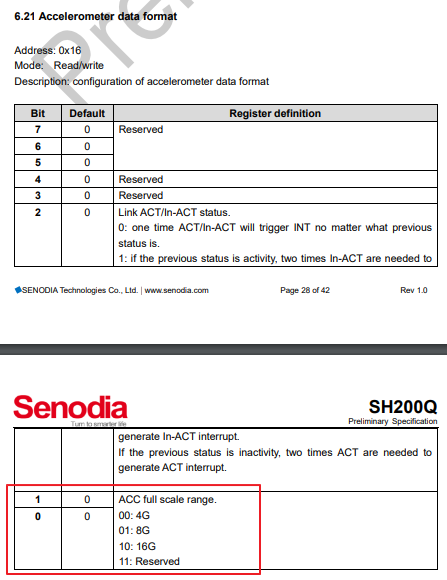# StickC - SH200Q Acceleration Range

• How to init the acceleration range to 16g for Sh200q on StickC with the MicroPython code?

• @zhufu86

I tried the code blow. But I don't know whether it is correct.

``````from hardware import sh200q
imu = sh200q.Sh200q(accel_fs=sh200q.ACCEL_FS_SEL_16G)
``````

• imu = sh200q.Sh200q(accel_fs=sh200q.ACCEL_FS_SEL_16G)

I tried the codes in the StickC and got the acceleration value four times as big as the value before. So I suppose SH200Q is initiated with 4g range if lack of accel_fs setting.
For temporary solution I devide the returned acceleration value by 4.

``````  x = imu.acceleration/4
y = imu.acceleration/4
z = imu.acceleration/4
``````

I also did some tests as below. No clue...

``````>>> imu = sh200q.Sh200q()
>>> imu._accel_so
8192
>>> imu.acceleration
0.995
>>> imu = sh200q.Sh200q(accel_fs=sh200q.ACCEL_FS_SEL_16G)
>>> imu._accel_so
2048
>>> imu.acceleration
4.058
>>> imu.acceleration/4
0.9985
>>> imu._accel_so=8192
>>> imu.acceleration
0.999
>>>
``````

• imu._accel_so=8192

Update...
No matter how I initiate SH200Q, I can not get the range larger than 4g.
Need more investigation.

• @zhufu86

It seems I need to write the correct configuration into the SH200Q register manually.Here is how to....

``````MicroPython ESP32_LoBo_v3.2.24 - 2018-09-06 on M5Stack with ESP32
>>> from hardware import sh200q
>>> imu = sh200q.Sh200q(accel_fs=sh200q.ACCEL_FS_SEL_16G)
>>> imu.acceleration
4.332
b'\x10'
>>> imu.i2c.writeto_mem(108,0x16,'\x12')
1
>>> imu.acceleration
1.086
>>>
``````

Is it a bug in module "hardware.sh200q"?

• ``````@property
def acceleration(self):
so = self._accel_so
data = self._regThreeShort(0x00)
return tuple([round(value / so, 3) for value in data])
``````

output data is not raw data, and ACCEL_FS_SEL_16G only measuring range, does not affect the value of acceleration

• I know, it a bug..., will fix it

• @zhufu86 Hey, I came along to look for python support for the sh200q. Seems that you use it in python with the hardware module.
May sound stupid but I'm unsure where to find the module... (Very new to m5Stick / m5Stack) I am using the m5Stick.

Best regards,
klaas